# Approximating the Set Cover ProblemI just finished my weekly task of shopping for groceries. This can be a somewhat daunting task because I generally have a list of things that I’ll need which cannot all be purchased at a single location. What often happens is that I find that many of the items on my list are ONLY offered at certain stores – generic brands of certain items for example. My goal then changes from minimizing the total amount of money spent to minimizing the number of stores that I must visit to purchase all of my items.

To formulate this as a mathematical problem, suppose that I have a grocery list of items I would like to buy, represented by the lists item1, item2, …, itemn, where n represents the number of items I have on this list. Suppose also that there are stores Store1, Store2, …, Storem (each one distinct) that offer some combination of items I have on my list. What I would like to do is minimize the number of stores I have to visit to purchase these items.

The problem I just described is famous because it is one that many people face on a regular basis. In a more general form, it is so famous that it has a name for it, called the Set Cover Problem (or the Minimum Set Cover Problem). In the general form of this problem, we replace the grocery list with a set of items called our universe. The lists of items offered at each store are the collections of subsets of the universe. In the problem, as in the example above, we would like to select enough subsets from this collection that we are able to obtain every element in our universe. We would like to do this with as low a number of sets as possible.

In my previous post, I described the 21 problems that Karp proved were NP-Complete. Set Cover was one of those problems, showing that this is a hard problem to solve. What I will do is introduce three ways to reach a near-optimal solution relatively quickly.

Greedy Method

One of the first approaches one may take to solve this problem is to repeatedly select the subset that contains the most new items. That’s how the greedy approach to set cover operates. The method knows to terminate when all elements belong to one of the selected sets. In the shopping example above, this would be accomplished by visiting the store that had the most items on my list and purchasing those items at this store. Once this is done, the items that have been purchased can be crossed off my list and we can visit the store with the most items on my remaining list, stopping when the list is empty.

Linear Programming Relaxation

Instead of stating the set cover problem with words, there is a way of describing the situation with mathematical inequalities. For instance, suppose that the soap I like to purchase is only available at stores Store1, Store4 and Store9. Then I could introduce a variable xi for each store i and the requirement that I purchase this soap can be restated as :

x1 + x4 + x9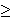1

Because we can either purchase some items or not purchase these items, each variable xi is 0 or 1 (called a binary variable). We can introduce similar constraints for each element in our universe (or on our grocery list). These inequalities (called constraints) have the form:

for each element e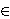U,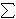i | eSi xi1

Our goal of minimizing the number of sets chosen (stores visited) can be stated by the objective function:
minimize1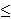in xi

So the mathematical formulation for this problem can be stated as

minimize1in xi
Subject to
for each element eU,i | eSi xi1
for each set i, xi{0, 1}.

Formulations of this type, where variables are restricted to a finite set (in this case the x variables being either 0 or 1) are called integer programs. Unfortunately, there is no easy way to solve these formulations either. However, there is a related problem which can be solved quickly.

Instead of restricting the x variables to the values of 0 or 1, we could allow them to take on any value within this range, i.e. 0xi1 for each set Si. Doing this converts the problem from an integer programming problem into a linear programming problem (called the LP-Relaxation), which can be solved quickly. The issue with this method though is that the solution obtained by an LP-Relaxation is not guaranteed to be an integer. In this case, how do we interpret the values xi?

Randomized Rounding Method

One approach to dealing with a non-integer solution to the LP-Relaxation is to treat the xi values as probabilities. We can say that xi is the probability that we select set i. This works because each value of xi is in the range of 0 to 1, which is necessary for a probability. We need to repeatedly select sets with their associated probabilities until all elements in our universe are covered. Selecting our sets based on this procedure is the randomized rounding approach.

Deterministic Rounding Method

A second approach to dealing with a non-integer solution to the LP-Relaxation is to base our solution on the most occurring element. If we let f be this frequency (i.e.the number of sets that the most occurring element occurs in), then we can define a solution by selecting set i if the LP=Relaxation solution gives the variable xi a value of at least (1/f).

None of these three approaches is guaranteed to give an optimal solution to an instance of this problem. I will not go into it in this post, but these can all be shown to be within some guaranteed range of the optimal solution, thus making them approximation algorithms.

Hope you enjoy.

# Learn About “the Other” AlgebraWhen I visit family for the holidays, the topic of my being a mathematician always seems to come up, and there’s always a child in the family struggling with maths, and when I ask the subject of their struggles the word “algebra” is always the culprit. I’ll save for another post my ideas on how this subject should be taught in high school and some of the main problems facing students.

I want to concentrate this post on a topic that few outside the mathematical world know about, but which many inside this world (myself included) hold dearly – the topic of modern or abstract algebra. I refer to this as “the other” algebra because a general conversation about the word “algebra” will generally revolve around concepts such as systems of equations, slopes, intercepts, intersection, rise-over-run, point-slope, and other terminology that limits algebra to a specific domain (the set of real or complex numbers) while at the same time ignoring the underlying beauty associated with this area.

I wrote previously about the area of set theory and the beauty associated with taking math out of the scope of a basic number line and into a much more undefined space. Abstract algebra is a continuation of set theory where in addition to our set, we have a (binary) operation defined on any two elements of this set. The inclusion of this binary operation allows us to consider several different structures based on the properties that this binary operation holds.

The structures I’d like to write about today are called groups. A group is a set along with an operation (or function) defined on any two elements of the set with the following properties:
– It is closed. This means that any time we run this function on two elements on the set, the function gives us a member of the set. In mathematical terms, for all a, b in the set A, f(a, b) must also be a member of A.
– There is an identity element. An identity element is defined as an element where is we include it in the binary operator with any other element, the operator will always return the other element. So if the element i is the identity element, then f(i, a) = a and f(a, i) = a for any other a in the set A. Any group must have an identity element.
– Every element has an inverse. Inverse elements are based on the identity element. What the inverse says is that for every element, there is a way to use the binary operator to get to the identity element. So for all elements a in the set A, there is an element b in the set A such that f(a, b) = i, where i is the identity element.
– The binary operator is associative. I described the associative property when I discussed the functions and relations of set theory. A function is associative if the way we group things (aka associate them) doesn’t matter. This means that for any elements a, b, and c of the set A, f(f(a, b), c) must be the same as f(a, f(b, c)).

If these four properties hold for a set A and a binary function f, then we say that the pair (A, f) is a group. We will generally use a common notation such as a · b, or a * b or simply ab to represent f(a, b).

Another important concept in group theory is the idea of a Cayley table. These are similar to multiplication tables that we drew out when we were first learning our “times tables”. For a group with n elements, we form a table with n rows and n columns. Each element of the group is written out to the left of each row and above each column (so really we can think of it as an n+1 by n+1 table with the first row and column being descriptive rows). Each cell of the table is the binary operator applied to the two elements indicated by the row and column (with an understanding of whether we have row before column or vice versa). Obviously, we can only do this for finite groups as we cannot write out all the elements of an infinite set.

The script I’ve added is a tester to allow users to input the information for a possible group (size, name of each element, and a Cayley table) and with this information the user is informed whether or not it forms a group. If it does not form a group, the reasons why it does not form one are also given. There are also some sample groups given to give insight into this area.

# Understanding Bayes’ Theorem

I’ve finished a script that helps understand Bayes’ Theorem.

If we have a set of mutually exclusive (aka non-overlapping) sets Bi for i{0, 1, 2, …, n} for some integer n, then the union of these sets forms a sample space. Lets call the sample space S. Suppose that we also have some set (also known as an event) A which is also a subset of S. Bayes’ Theorem considers the probability that one of these mutually exclusive events (one of the Bi‘s) caused the observed event (A).

This probability can be calculated by the formula

 Pr(Bj | A) = Pr(Bj) Pr(A | Bj)Pr(Bi) Pr(A | Bi)

The theorem helps us determine the the probability of the event Bj given A, or in more plain English, the probability that the event Bj is the cause that gives rise to the observed event A. The numerator is given by the product of of the probability of the causal event (Pr(Bj) times the conditional probability of the observed event given the causal event (Pr(A | Bj)). This numerator could be replaced by its equivalent statement of the set A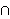Bj. Likewise, the denominator the sum (over all the causal events) of the probaility of each causal event times the conditional probability of the observed event given that particular causal event. Each term in this denominator could be replaced b its equivalent staetment ABi, which when summed give the total probability of A because each pair of the Bj‘s is mutually exclusive. So we are able to replace the probability of A withPr(Bi) Pr(A | Bi) because of the fundamental law of probability.

An example that would use Bayes’ Theorem is analyzing the results of an election. The set of mutually exclusive events could be membership in a political party (Democrat, Republican, or Independent). The observed event could be the election of an individual. And the conditional distributions could be the percentage of each party that voted for this individual. If we want to calculate how significant each party was to the individual’s election, we’d use Bayes’ Theorem.

The script I’ve written to help understand Bayes’ Theorem works as follows:
– A set of mutually exclusive sets is randomly generated (the number of sets also varies). These sets are called Bi for i(0, …, n}.
– A set A is randomly generated from the union of the Bi‘s.
– A table is displayed showing:
Pr(Bi) for each i on line 1.
Pr(A | Bi) for each i on line 2.

– The user is given the option to select which of the mutually exclusive sets they would like to use to calculate the probability that this set caused the event A.
– Once a set is chosen, the user clicks the “Calculate Conditional” button and Bayes’ Theorem gives the result.
– If the “show work” checkbox was checked, then the steps used in this calculation are also shown.
– All work is done using fractions to give an idea of where the numbers come from.

Other Blogs that have covered this topic:
Better Explained
Bayes’ Theorem-qed

# Learn Math Through Set RelationsMuch of our world deals with relationships – both in the sense of romantic ones or ones that show some interesting property between two sets. When mathematicians think of set theory, a relation between the set A and the set B is a set of ordered pairs, where the first element of the ordered pair is from the set A and the second element of the ordered pair id from the set B. So if we say that R is a relation on the sets A and B, that would mean that R consists of elements that look like (a, b) where a is in A and b is in B. Another way of writing this is that R is a subset of A x B. For more on subsets and cross product, I refer you to my earlier script work on set operations.

Relations can provide a useful means of relating an abstract concept to a real world one. I think of things like the QB rating system in the NFL as an example. We have a set of all quarterbacks in the NFL (or really all people who have thrown a pass) and we would like some means of saying that one QB is performing better than another. The set of statistics kept on a QB is a large set, so attempting to show that one QB is better by showing that every year that they played one is better in every statistical category can be (a) exhaustive, and (b) will lead to very few interesting comparisons. Most of the really good QBs have some areas that they are really good and others that they are not. The QB rating system provides a relation between the set of all QBs in the NFL and the set of real numbers. Once this relation was defined, we can say that one QB is performing better than another if his QB rating is higher. Similarly we can compare a QB to his own statistics at different points in his career to see the changes and trends.

This is just one example, and there are countless others that I could have used instead.

Once we understand what a relation is, we have several properties that we are interested in. Below I list four, although there are many more.

Properties of Relations:
A relation R is symmetric if whenever an element (a, b) belongs to R, then so does (b, a).

A relation R is reflexive if for every element a in the universe of the relation, the element (a, a) belongs to R.

A relation R is transitive if for every pair of elements (a, b) and (c, d) and b = c, then the element (a, d) belongs to R.

A relation R is anti-symmetric if the elements (a, b) and (b, a) do not belong to the relation whenever a is not equal to b.

Once we understand what a relation is, there are a few common ones that we are interested in. Below I list four, but again, I want to stress that these are some of the more common ones, but there are several others.

Types of Relations:
A relation R is a function (on its set of defined elements) if there do not exist elements (a, b) and (a, c) which both belong to R.

A relation R is an equivalence relation if R is symmetric, reflexive and transitive.

A relation R is a partial order set if R is anti-symmetric, reflexive and transitive.

A relation R is a total order set if it is a partial order set and for every pair of elements a and b, either (a, b) is in R or (b, a) is in R.

A partial order is just an ordering, but not everything can be compared to everything else. Think about the Olympics, and a sport like gymnastics. Consider the floor and the balance beam. One person can win gold on the floor and another person wins gold on the balance beam. That puts each of them in the “top” of the order for their particular section, but there’s no way of comparing the person who won the floor exercise to the person who won the balance beam. So we say the set is “partially ordered”. More formally, lets say that two people (person X and person Y) relate if they competed in the same event and the the first person (in this case person X) received an equal or higher medal in that event than the second person (in this case person Y). Obviously any person receives the same medal as themselves, so this relation is reflexive. And if Jamie received an equal or higher medal than Bobby and Bobby received an equal or higher medal than Chris, then Jamie must have received an equal or higher medal than Chris so this relation is transitive. To test this relation for anti-symmetry, suppose that Chuck received an equal or higher medal than Charlie and Charlie received an equal or higher medal than Chuck. This means that they must have received the same medal, but since only one medal is awarded at each color for each event (meaning one gold, one silver and one bronze…if this is not true, assume it is), this must mean that Chuck and Charlie are the same person, and this relation is thus anti-symmetric.

If we have a partial ordering where we can compare everything, then we say that the set is “totally ordered”.

An equivalence relation tries to mimic equality on our relation. So, staying with that example of the Olympics, an example of an equivalence relation could be to say that two athletes relate to one another if they both received the same color medal in their event (for the sake of argument lets assume that no athlete competes in more than one event). Then obviously an athlete receives the same medal as themselves, so this relation is reflexive. If two people received the same medal, then it doesn’t matter if we say Chris and Charlie or Charlie and Chris, so the relation is symmetric. And Finally if Chris received the same medal as Charlie an if Charlie received the same medal as Jesse, then all three people received the same medals, so Chris and Jesse received the same medals and this relation is transitive. Because this relation has these three properties, it is called an equivalence relation.

# Learning Math through Set Theory

In grade school, we’re taught that math is about numbers. When we get to college (the ones of us who are still interested in math), we’re taught that mathematics is about sets, operations on sets and properties of those sets.

Understanding Set Theory is fundamental to understanding advanced mathematics. Iv wrote these scripts so that users could begin to play with the different set operations that are taught in a basic set theory course. Here, the sets are limited to positive integers and we’re only looking at a few operations, in particular the union, intersection, difference, symmetric difference, and cross product of two sets. I will explain what each of these is below.

The union of the sets S1 and S2 is the set S1 [union] S2, which contains the elements that are in S1 or S2 (or in both).
Note: S1 [union] S2 is the same as S2 [union] S1.

The intersection of the sets S1 and S2 is the set S1 [intersect] S2, which contains the elements that are in BOTH S1 and S2.
Note: S1 [intersect] S2 is the same as S2 [intersect] S1.

The difference between the sets S1 and S2 is the set S1 / S2, which contains the elements that are in S1 and not in S2.
. Note. S1 / S2 IS NOT the same as S2 / S1.
Note. S1 / S2 is the same as S1 [intersect] [not]S2.

The symmetric difference between the sets S1 and S2 is the set S1 [symm diff] S2, which contains the elements that are in S1 and not in S2, or the elements that are in S2 and not in S1.
Note. S1 [symm diff] S2 is the same as S2 [symm diff] S1.
Note. S1 [symm diff] S2 is the same as (S1 [intersect] [not] S2) [union] (S2 [intersect] [not] S1).

The cartesian product of the two sets S1 and S2 is the set of all ordered pairs (a, b), where a [in] S1 and b [in] S2.